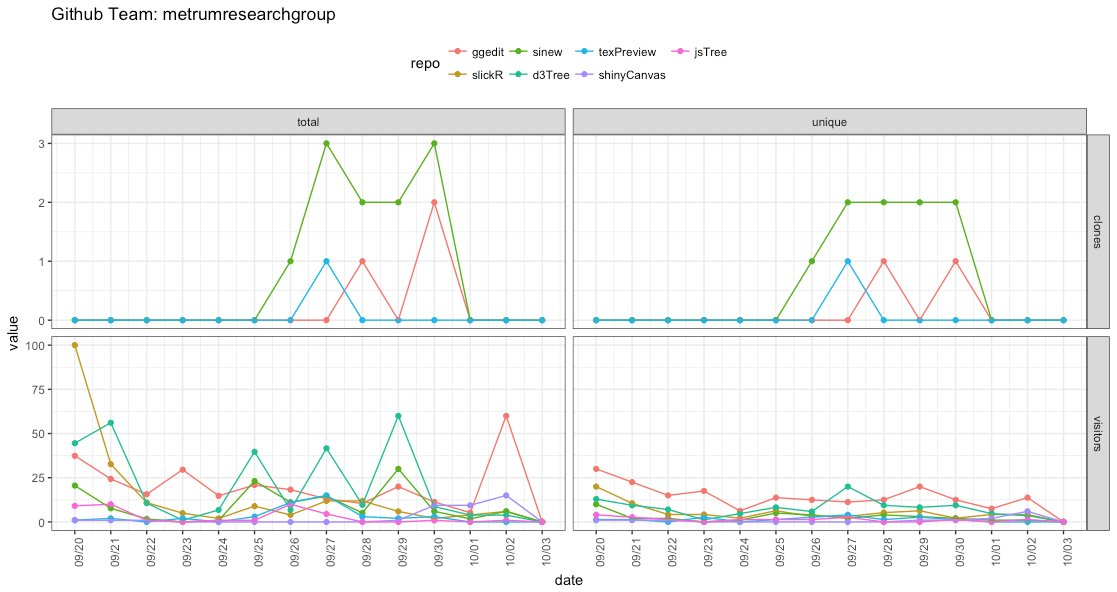Want to share your content on R-bloggers? click here if you have a blog, or here if you don't.

This post will show how to use the RSelenium package to scrape your own github account to retrieve all that fun traffic data of clones and visits and create a single traffic plot for your account.For the single file you can find it in this gist file.

## Packages

```library(RSelenium)
library(XML)
library(ggplot2)
library(reshape2)
library(plyr)
library(dplyr)
```

Fill in the relevant information for your account. The team is usually your username, but it can be different. The repos can be a vector and since we are going in the front door of the site we can access the private repositories too!

## Setup

```gh_user <- ''
gh_pass <- ''

gh_team <- ''
repos <- ''
```

## The function

```github_traffic <- function(gh_user,gh_pass,gh_team,repos){

#open the connection

rD <- RSelenium::rsDriver(verbose = FALSE)
remDr <- rD[["client"]]

#going to the first repo to invoke the login

remDr\$navigate(sprintf('https://github.com/%s/%s/graphs/traffic',gh_team,repos))

#entering the login information in the form and clicking the button.

webElem <- remDr\$findElement(using = 'id', value = "login_field")
webElem\$setElementAttribute(attributeName = 'value',value = gh_user)
webElem <- remDr\$findElement(using = 'id', value = "password")
webElem\$setElementAttribute(attributeName = 'value',value = gh_pass)
webElem\$clickElement()
Sys.sleep(1)

# Retrieve the plots into an html
out <- plyr::llply(repos,function(repo){
remDr\$navigate(sprintf('https://github.com/%s/%s/graphs/traffic',gh_team,repo))
Sys.sleep(1)
out <- XML::htmlParse(remDr\$getPageSource(),asText = TRUE)
sapply(c('clones','visitors'),function(type){
XML::getNodeSet(out,sprintf(sprintf('//*[@id="js-%s-graph"]/div/div/svg/g/g',type)))
},simplify = FALSE,USE.NAMES = TRUE)
},.progress = 'text')

# set the names (llply doesnt)
names(out) <- repos

# that's it we dont need the connection anymore
remDr\$close()
rD[["server"]]\$stop()

# scrape the data from html into a data.frame

plot_data <- plyr::ldply(out,function(repo){
plyr::mdply(names(repo),function(type){

dat <- repo[[type]]

if(is.null(dat)) return(NULL)

# tick values we need for rescaling
yticks_total <- as.numeric(sapply(getNodeSet(dat[],'g'),XML::xmlValue))
yticks_unique <- as.numeric(sapply(getNodeSet(dat[],'g'),XML::xmlValue))

x <- data.frame(type=type,
date = as.Date(sapply(getNodeSet(dat[],'g'),XML::xmlValue),format = '%m/%d'),
total = as.numeric(sapply(getNodeSet(dat[],'circle'),XML::xmlGetAttr,name = 'cy')),
unique = as.numeric(sapply(getNodeSet(dat[],'circle'),XML::xmlGetAttr,name = 'cy')))

# Because this is a d3.js object there are some technical details that
# I'm skipping here, but in short the y values need to be rescaled
# to show the actual values that you need.
x\$total <- scales::rescale(x\$total,rev(range(yticks_total)))
x\$unique <- scales::rescale(x\$unique,rev(range(yticks_unique)))

#rehape the data.frame from wide to long
x%>%reshape2::melt(.,c('type','date'),variable.name=c('metric'))
})
},.id='repo')

#Create the plot

ggplot(plot_data,aes(x=date,y=value,colour=repo))+
geom_point()+geom_line()+
facet_grid(type~metric,scales='free_y')+
scale_x_date(date_breaks = "1 day",date_labels = "%m/%d")+
theme_bw()+
theme(axis.text.x = element_text(angle=90),legend.position = 'top')+
labs(title=sprintf('Github Team: %s',gh_team))
}
```

## Run the function

```traffic_plot <- github_traffic(gh_user=gh_user,
gh_pass=gh_pass,
gh_team=gh_team,
repos=repos)
```
```traffic_plot
```If the function fails for some reason this will release the port `RSelenium` is holding ransom.

```rD <- RSelenium::rsDriver(verbose = FALSE,port=4444L)
remDr <- rD\$client
remDr\$close()
```